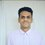# Doubt on integration

How to solve this question $\int\limits_0^1\frac{x^{2}+x+1}{x^{4}+x^{3}+x^{2}+x+1}dx$. Please help me in solving this.5 years, 11 months ago

This discussion board is a place to discuss our Daily Challenges and the math and science related to those challenges. Explanations are more than just a solution — they should explain the steps and thinking strategies that you used to obtain the solution. Comments should further the discussion of math and science.

When posting on Brilliant:

• Use the emojis to react to an explanation, whether you're congratulating a job well done , or just really confused .
• Ask specific questions about the challenge or the steps in somebody's explanation. Well-posed questions can add a lot to the discussion, but posting "I don't understand!" doesn't help anyone.
• Try to contribute something new to the discussion, whether it is an extension, generalization or other idea related to the challenge.

MarkdownAppears as
*italics* or _italics_ italics
**bold** or __bold__ bold
- bulleted- list
• bulleted
• list
1. numbered2. list
1. numbered
2. list
Note: you must add a full line of space before and after lists for them to show up correctly
paragraph 1paragraph 2

paragraph 1

paragraph 2

[example link](https://brilliant.org)example link
> This is a quote
This is a quote
    # I indented these lines
# 4 spaces, and now they show
# up as a code block.

print "hello world"
# I indented these lines
# 4 spaces, and now they show
# up as a code block.

print "hello world"
MathAppears as
Remember to wrap math in $$ ... $$ or $ ... $ to ensure proper formatting.
2 \times 3 $2 \times 3$
2^{34} $2^{34}$
a_{i-1} $a_{i-1}$
\frac{2}{3} $\frac{2}{3}$
\sqrt{2} $\sqrt{2}$
\sum_{i=1}^3 $\sum_{i=1}^3$
\sin \theta $\sin \theta$
\boxed{123} $\boxed{123}$

Sort by:

Multiply top and bottom by $-(x-1)$, we have $\displaystyle \int_0^1 \frac{1-x^3}{1-x^5} \, dx$.

Notice that the integrand can be written as a sum of a convergent geometric series with common ratio $x^5$.

\begin{aligned} \displaystyle \int_0^1 \frac{1-x^3}{1-x^5} \, dx &=& \displaystyle \int_0^1 \displaystyle \sum_{r=0}^\infty (1-x^3) x^{5r} \, dx \\ \displaystyle &=& \sum_{r=0}^\infty \int_0^1 (x^{5r} - x^{3+5r} ) \, dx \\ \displaystyle &=& \sum_{r=0}^\infty \left( \frac1{5r+1} - \frac1{5r+4}\right ) \\ \displaystyle &=& \sum_{r=0}^\infty \frac3{25r^2+25r+4} \qquad (\star) \\ \end{aligned}

We consider the logarithmic differentiation of the Weiestrass Product:

$\displaystyle \cos(\pi x) = \prod_{n=0}^\infty \left( 1 - \frac{4x^2}{(2n+1)^2} \right)$

to get

$\displaystyle \sum_{n=0}^\infty \frac1{(2n+1)^2 - (2x)^2} = \frac{\pi}{8x} \tan(\pi x ) \qquad (\star \star)$

Notice that $\frac3{25r^2+25r+4} = \frac{12}{25} \cdot \frac1{(2r+1)^2 - \left (2\cdot \frac3{10} \right)^2}$. Thus $x=\frac3{10}$ for $(\star \star)$.

Hence, the integral in question equals to $\dfrac{12}{25} \cdot \dfrac{\pi}{8\cdot 3/10} \tan\left(\pi \cdot \dfrac3{10}\right) = \dfrac\pi5 \tan\left(\dfrac3{10}\pi\right)$.

Now, we just need to evaluate $\tan\left(\frac3{10}\pi\right)$. Apply the identity $\tan(x) = \sqrt{\frac{1-\cos(2x)}{1+\cos(2x)}}$ for $x = \frac3{10}\pi$.

This means we need to find what is the value of $\cos\left(\frac35\pi \right)$. Let $y$ denote this value. Because $\cos\left(\frac{1}5\pi\right) = -\cos\left( 4\times \frac15\pi \right)$. Apply the double angle formula twice yields $\cos\left( \frac15\pi\right) = \frac{1+\sqrt5}4$. Apply the triple angle formula to get $y = \frac{1-\sqrt5}4$.

Substitution yields the answer of $\displaystyle \pi \sqrt{\dfrac{5+2\sqrt5}{125}} \approx 0.8648 \ \square$

- 5 years, 11 months ago

Is it correct to switch the integration and summation?

- 5 years, 11 months ago

Yes, apply Fubini's (or Tonelli's) theorem.

- 5 years, 11 months ago

Can u please lend me ur brains for giving jee. Lol i was just kidding. Amazing sol.

- 5 years, 11 months ago

Great solution. Thanks.

- 5 years, 11 months ago

What makes you think that it has an exact form?

- 5 years, 11 months ago

This question was given to me in my classes and was also told that answer was in terms of pi.

- 5 years, 11 months ago

@Shivam Jadhav divide the denominator by the numerator. Then u can split it up into partial fractions. Then u can proceed it using normal methods

- 5 years, 11 months ago

Wait what? How is that possible? Doesn't it complicate things?

- 5 years, 11 months ago

yes it actually complicates it.

- 5 years, 11 months ago

Can you please solve it for me?

- 5 years, 11 months ago

- 5 years, 11 months ago

Check out the Integration of Rational Functions that @Pranshu Gaba and @Vishnuram Leonardodavinci have been working on.

Staff - 5 years, 11 months ago

Answer is $\pi \sqrt{\dfrac{5+2\sqrt5}{125}} \approx 0.8648$. I will post the solution shortly.

- 5 years, 11 months ago

Hint: Factorize the denominator into two irreducible quadratic equations.

- 5 years, 11 months ago